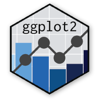Show Sidebar Hide Sidebar# scale_x_date in ggplot2

How to make plots in R and ggplot2 using scale_x_date.

### New to Plotly?

Plotly's R library is free and open source!
You can set up Plotly to work in online or offline mode.
We also have a quick-reference cheatsheet (new!) to help you get started!

### Version Check

Version 4 of Plotly's R package is now available!
Check out this post for more information on breaking changes and new features available in this version.

library(plotly)
packageVersion('plotly')

##  '4.9.1'


## By Month

library(plotly)
library(scales)

x <- c("04-01-10","05-01-10","06-01-10","07-01-10","08-01-10","09-01-10","10-01-10","11-01-10","12-01-10","01-01-11","02-01-11","03-01-11","04-01-11","05-01-11","06-01-11","07-01-11","08-01-11","09-01-11","10-01-11","11-01-11","12-01-11","01-01-12","02-01-12","03-01-12","04-01-12","05-01-12","06-01-12")
y <- c(120,210,130,160,190,210,80,70,110,120,140,160,130,200,110,180,210,200,90,60,100,100,120,170,100,180,120)

MySample <- data.frame(x) ## convert to dataframe
MySample$y <- y # install.packages("lubridate") require(lubridate) MySample$date <- as.Date(MySample$x, "%m-%d-%y") MySample$year <- year(MySample$date) p <- ggplot(MySample, aes(date, y, fill = year)) + geom_bar(stat="identity") + facet_grid(. ~ year, scales = "free") + scale_x_date(labels = date_format("%b/%y")) + scale_fill_gradient(breaks=unique(MySample$year))

p <- ggplotly(p)

p


Inspired by Stack Overflow.

## By Year

library(plotly)
library(scales)

set.seed(12345)
Date <- seq(as.Date("2010/1/1"), as.Date("2014/1/1"), "week")
Y <- rnorm(n=length(Date), mean=100, sd=1)
df <- data.frame(Date, Y)

df$Year <- format(df$Date, "%Y")
df$Month <- format(df$Date, "%b")
df$Day <- format(df$Date, "%d")

df$MonthDay <- format(df$Date, "%d-%b")

df$CommonDate <- as.Date(paste0("2000-",format(df$Date, "%j")), "%Y-%j")

p <- ggplot(data = df,
mapping = aes(x = CommonDate, y = Y, shape = Year, colour = Year)) +
geom_point() +
geom_line() +
facet_grid(facets = Year ~ .) +
scale_x_date(labels = function(x) format(x, "%d-%b"))

p <- ggplotly(p)

p


Inspired by Stack Overflow.